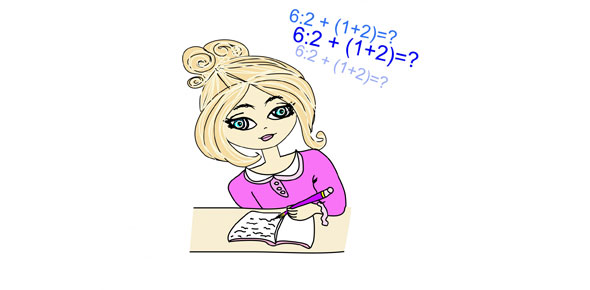# SAT Section 4 Math

16 Questions | Total Attempts: 58SettingsThis is Section 4 of the SAT. You are allowed to use a calculator, but it is not required. Fill in the bubble for the best answer to each question.

• 1.
If a² = 12, then a4 = ?
• A.

144

• B.

72

• C.

36

• D.

24

• E.

16

• 2.
A dress on sale in a shop is marked at \$D. During the discount sale its price is reduced by 15%. Staff are allowed a further 10% reduction on the discounted price. If a staff member buys the dress what will she have to pay in terms of D?
• A.

0.75D

• B.

0.76D

• C.

0.765D

• D.

0.775D

• E.

0.805D

• 3.
A certain animal in the zoo has consumed 39 pounds of food in six days. If it continues to eat at the same rate, in how many more days will its total consumption be 91 pounds?
• A.

12

• B.

11

• C.

10

• D.

9

• E.

8

• 4.
If n is even, which of the following cannot be odd?I n + 3II 3nIII n² - 1
• A.

I only

• B.

II only

• C.

III only

• D.

I and II only

• E.

I, II, and III

• 5.
One side of a triangle has length 8 and a second side has length 5. Which of the following could be the area of the triangle?I 24II 20III 5
• A.

I only

• B.

II only

• C.

III only

• D.

II and III only

• E.

I, II, and III

• 6.
N is an integer chosen at random from the set{5, 7, 9, 11 }p is chosen at random from the set{2, 6, 10, 14, 18}What is the probability that n + p = 23 ?
• A.

0.1

• B.

0.2

• C.

0.25

• D.

0.3

• E.

0.4

• 7.
If the radius of the circle with centre O is 7 and the measure of angle AOB is 100, what is the best approximation to the length of arc AB ?
• A.

9

• B.

10

• C.

11

• D.

12

• E.

13

• 8.
Sheila works 8 hours per day on Monday, Wednesday and Friday, and 6 hours per day on Tuesday and Thursday. She does not work on Saturday and Sunday. She earns \$324 per week. How much does she earn in dollars per hour?
• A.

11

• B.

10

• C.

9

• D.

8

• E.

7

• 9.
If the product of 6 integers is negative, at most how many of the integers can be negative?
• A.

2

• B.

3

• C.

4

• D.

5

• E.

6

• 10.
If a positive integer n, divided by 5 has a remainder 2, which of the following must be true? I n is odd II n + 1 cannot be a prime number III (n + 2) divided by 7 has remainder 2
• A.

None

• B.

I only

• C.

I and II only

• D.

II and III only

• E.

I, II, and III

• 11.
A solid cube of side 6 is first painted pink and then cut into smaller cubes of side 2. How many of the smaller cubes have paint on exactly 2 sides?
• A.

30

• B.

24

• C.

12

• D.

8

• E.

6

• 12.
Line l contains the points (3,1) and (4,4). If line m is a different line, parallel to line l in the same coordinate plane, which of the following could be the equation of line m?
• A.

Y = 3x - 8

• B.

Y = 1/3x - 3

• C.

Y = -3x - 8

• D.

Y = 3x + 1

• E.

Y = -8x + 3

• 13.
A triangle has a perimeter 13. The two shorter sides have integer lengths equal to x and x + 1. Which of the following could be the length of the other side?
• A.

2

• B.

4

• C.

6

• D.

8

• E.

10

• 14.
A machine puts c caps on bottles in m minutes. How many hours will it take to put caps on b bottles?
• A.

60bm/c

• B.

Bm/60c

• C.

Bc/60m

• D.

60b/cm

• E.

B/60cm

• 15.
Paint needs to be thinned to a ratio of 2 parts paint to 1.5 parts water. The painter has by mistake added water so that he has 6 liters of paint which is half water and half paint. What must he add to make the proportions of the mixture correct?
• A.

1 liter paint

• B.

1 liter water

• C.

½ liter water and one liter paint

• D.

½ liter paint and one liter water

• E.

½ liter paint

• 16.
6 pints of a 20 percent solution of alcohol in water are mixed with 4 pints of a 10 percent alcohol in water solution. The percentage alcohol in the new solution is ___.
• A.

16

• B.

15

• C.

14

• D.

13

• E.

12

Related TopicsBack to top